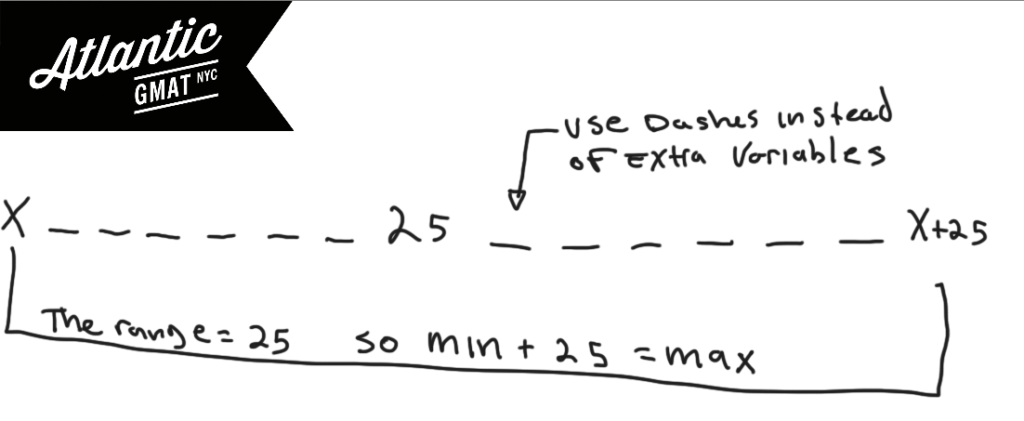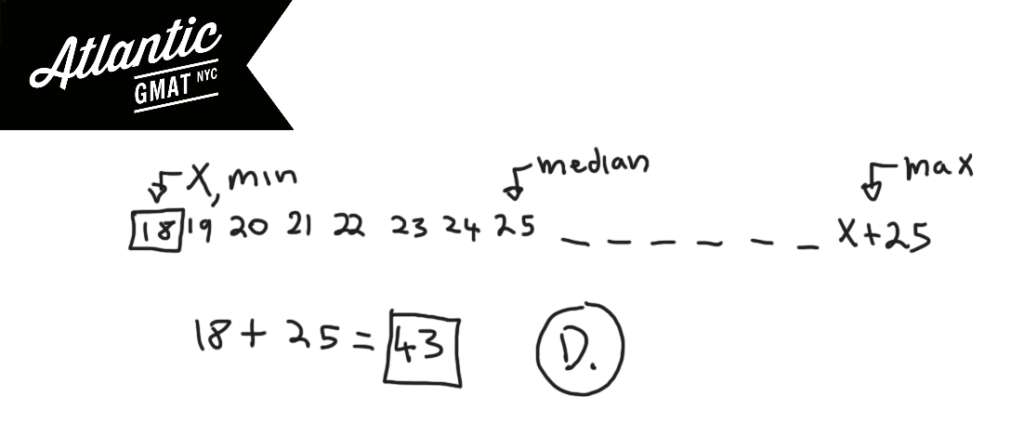A set of 15 different integers has median of 25 and a range of 25. What is greatest possible integer that could be in this set?

(A) 32
(B) 37
(C) 40
(D) 43
(E) 50

This GMAT question falls firmly into statistics. Foggy on median and range? Go back to the GMAT basics! Get this fundamental GMAT content down backwards and forwards so that you can focus on the critical thinking portion of the exam. Median is the middle number of an odd set or the average of the two middle numbers of an even set. Range is the biggest number minus the smallest number (Range = max - min). Let's start by plotting out what we know. Median is spatial so demands a diagram. In a set of 15 numbers the median is the 8th number (7 above it, 7 below it). Especially for statistics set questions avoid creating a million variables for numbers you're not solving for. Let's represent those numbers with dashes and create a variable for the min. Then you can add 25 to that for the max. Keep in mind what we're solving for: the greatest possible integer that could be in this set.We know the range is 25 and that we want to make the max as big as possible. So what should we do with the min? We want to make the min as big as possible so that min + 25 (the max) is as big as possible. You think: let's make the min = median. Good thinking! That would be perfect. But that's not possible as it is a set of 15 different integers. Still, we're on the right track. Let's just go down as little as possible from the median. 24.9999999999. Good thinking again! But, we have to stick with integers. So then you've got 24, 23, 22, 21, 20, 19, 18. Add 25 to 18 and that's the greatest possible integer in the set. Comment with any questions or additions. Happy GMAT studying!# CONTACT

Atlantic GMAT

405 East 51st St.

NY, NY 10022

(347) 669-3545

info@AtlanticGMAT.com